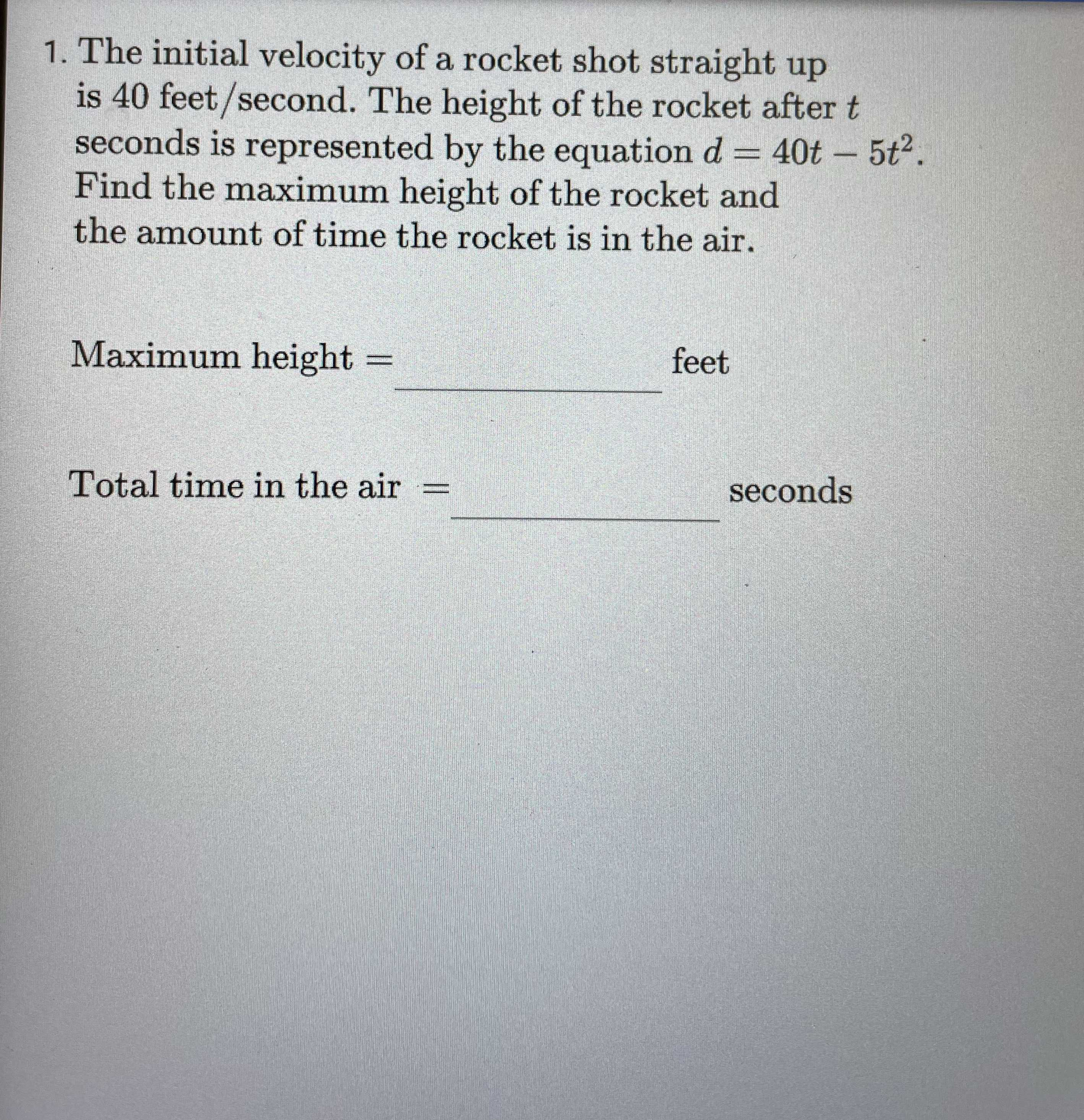### ¿Todavía tienes preguntas de matemáticas?

Pregunte a nuestros tutores expertos
Algebra
Pregunta1. The initial velocity of a rocket shot straight up is $$40$$ feet/second. The height of the rocket after $$t$$ seconds is represented by the equation $$d = 40 t - 5 t ^ { 2 }$$ . Find the maximum height of the rocket and the amount of time the rocket is in the air. Maximum height $$=$$ Total time in the air $$=$$